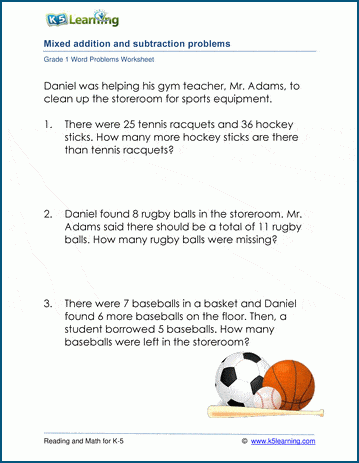# 1st Grade Math Word Problems Worksheets

These grade 1 word problem worksheets relate first grade math concepts to the real world. The word problems cover addition, subtraction, time, money, fractions and lengths.

We encourage students to think about the problems carefully by:

• providing a number of mixed word problem worksheets;
• sometimes including irrelevant data within word problems.

Addition with sums 50 or less

3 or more numbers added together

## Subtraction word problems

Subtracting single digit numbers

Subtracting numbers under 50

## Mixed addition and subtraction word problems

Add / subtract word problems with mostly single digit numbers

Add / subtract word problems with numbers under 50

## Time word problems

Time and elapsed time problems (whole hours)

## Money word problems

Counting money (coins only)

## Measurement word problems

Combining and comparing lengths (inches)

Combining and comparing lengths (cm)

## Fraction word problems

Write the fraction from the story (parts of whole, parts of group)

## Mixed word problemsSample Grade 1 Word Problem Worksheet

## More word problem worksheets

Explore all of our math word problem worksheets, from kindergarten through grade 5.

What is K5?

K5 Learning offers free worksheets, flashcards and inexpensive workbooks for kids in kindergarten to grade 5. Become a member to access additional content and skip ads.